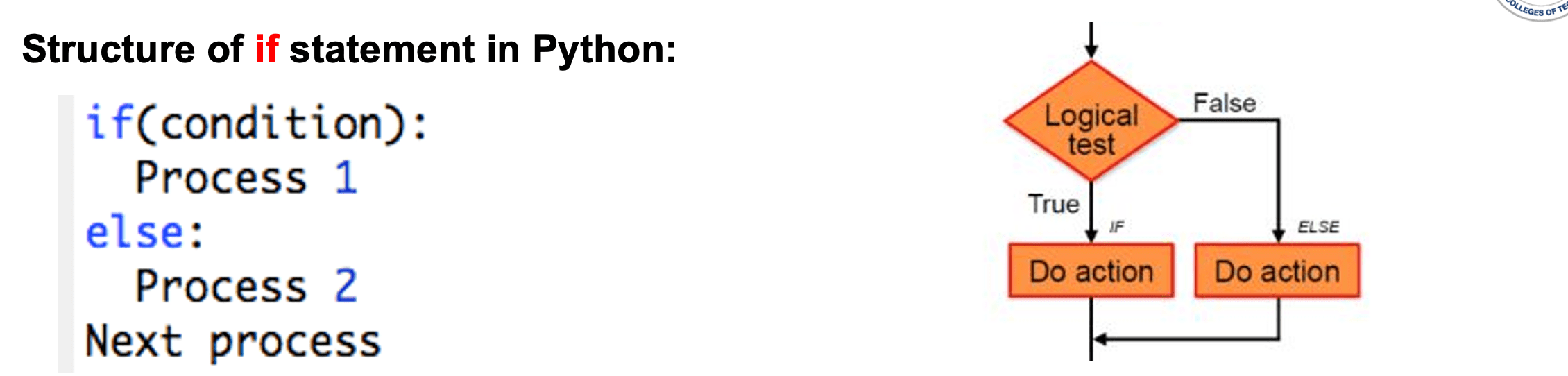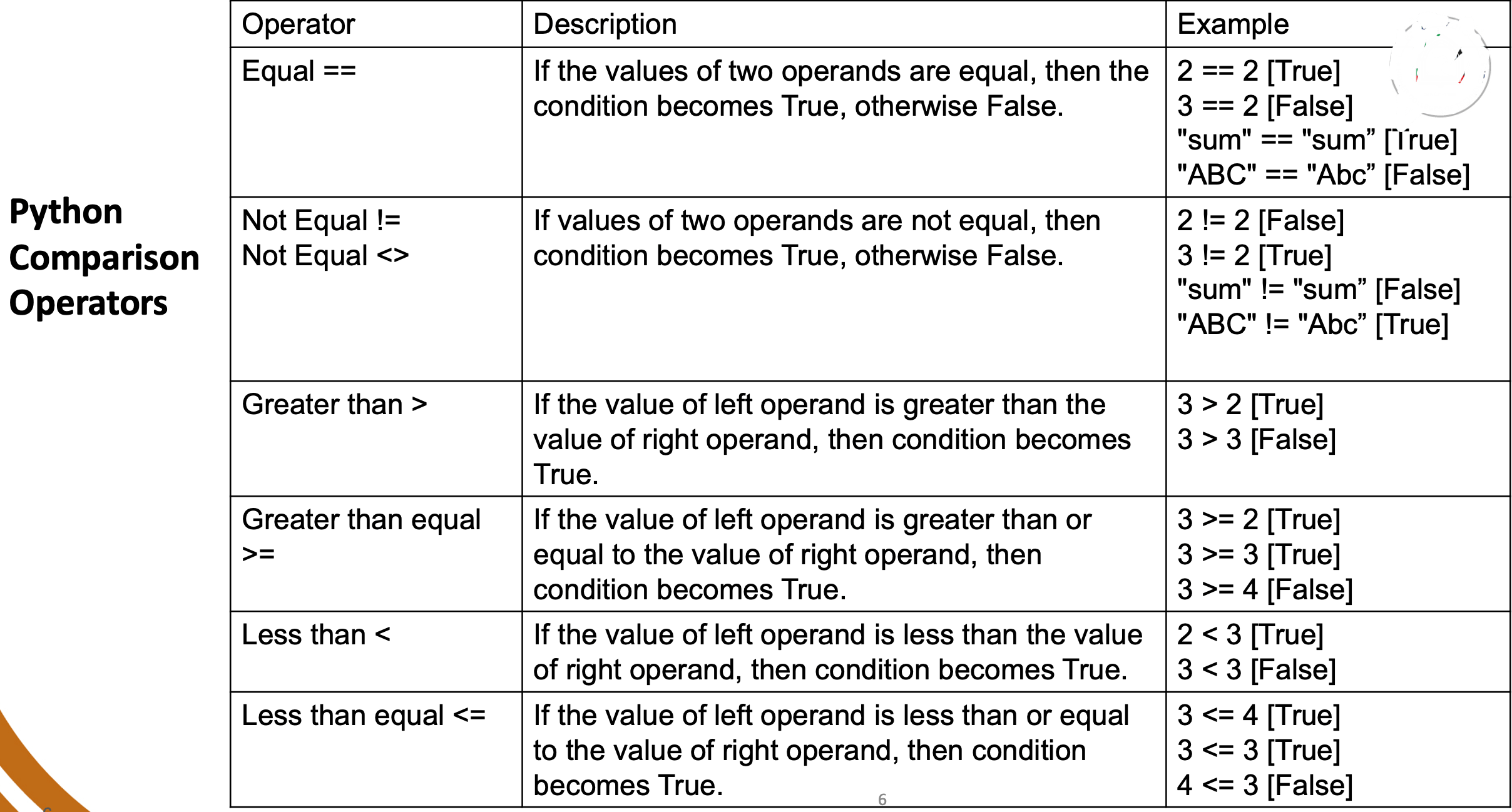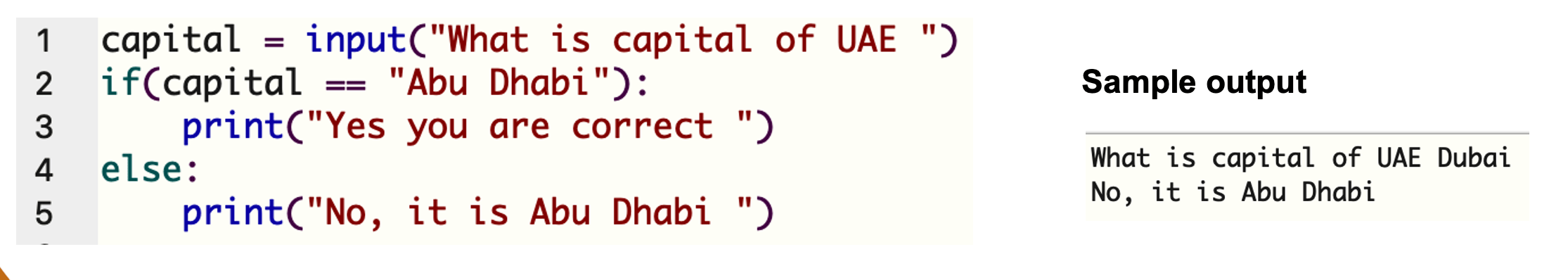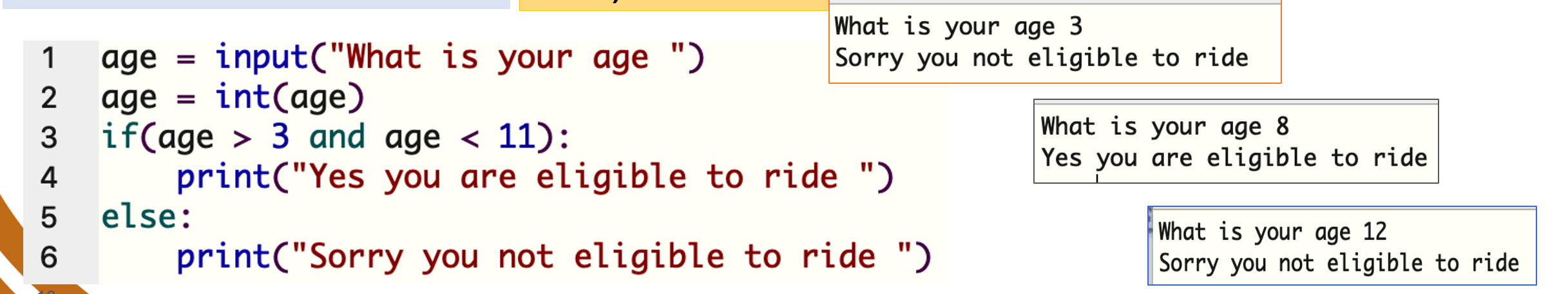# Selections

## Selections: if statement

Example: " If it is raining outside, then I carry umbrella."

In programming such logic is written using if statement.

• If the answer to the if statement is True, then a certain process will be executed, else other processes will be executed.
• Python programming language provides following types of decision making statements.
• If..else
• nested if
• nested else ifRules:
• A lower case if keyword must be used
• The if statement must end with a colon :
• The keyword else must be lower case and start at the same level as the keyword if
• The keyword else must end with a colon :### Example 1 ( Condition with numeric )

```        emirates = input(" How many emirates are in UAE? ")
emirates = int(emirates)
if(emirates == 7):
print(" Yes you are correct ")
else:
print(" No, there are 7 emirates ")
```
Here is the code and its output:### Example 2 ( Condition with non numeric)

```	capital = input(" What is capital of UAE? ")
if(capital == " Abu Dhabi"):
print(" Yes you are correct ")
else:
print(" No, it is Abu Dhabi ")
```
Here is the code and its output:Note: String comparision is case sensitive.

### Example 3 ( Condition with non numeric )

Write a Python program to calculate the cost of insurance based on the age. If the age of the subscriber is more than or equal 40 years, the cost will be 500 each month, otherwise the cost is 300 a month.

```	    age = input (" What is your age? ")
age = int(age)

insurance = 0

if (age >= 40):
insurance = 500
else:
insurance = 300

print (insurance)
```

## Logical Operators

When you have more than one condition in the same if statement [compound condition], then you need to use a logical operator. These logical operators simply allow you to request that both conditions must be met or only one of them.

• If both are conditions must be True then use and
• If Any one of the conditions is True then use or
 C1 C2 C1 and C2 C1 or C2 false false false false false true false true true false false true true true true true

 Operator Description Example and If both the operands are true then condition becomes true. 3 >7 and 2<3 or If any of the two operands are non-z ero then condition becomes true 7 > 7 or 2 < 3

## Logical Operators ( 2 )

When you have more than one condition, it is recommended to group them using brackets.
Think of a situation when condition 1 to be met and one of the other two conditions

```	        if (condition1) and (condition2 or condition3):
Process1
else:
Process2
Nextprocess
```

### Example 4 ( Condition with non numeric)

A child is eligible to enter a ride if his/her age is between 4 to 10. Write a Python program to read child’s age, decide and display if the child is eligible for ride or not.

```	age= input("What is your age? ")
age= int(age)

if(age > 3 and age < 11):
print(" Yes you are eligible to ride " )
else:
print ("Sorry you are not eligible to ride ")

```
Here is the code and its output: